林业科学2016, Vol. 52Issue (11): 115-123PDF
DOI: 10.11707/j.1001-7488.20161114
0

#### 文章信息

You Lei, Tang Shouzheng, Song Xinyu

An Algorithm of Stem Surface Reconstruction Based on Tangent Plane Projection

Scientia Silvae Sinicae, 2016, 52(11): 115-123.
DOI: 10.11707/j.1001-7488.20161114

### 作者相关文章

1. 信阳师范学院计算机与信息技术学院 信阳 464000;
2. 中国林业科学研究院资源信息研究所 北京 100091;
3. 信阳师范学院数学与信息科学学院 信阳 464000

An Algorithm of Stem Surface Reconstruction Based on Tangent Plane Projection
You Lei1,2, Tang Shouzheng2, Song Xinyu3
1. College of Computer and Information Technology, Xinyang Normal University Xinyang 464000 ;
2. Research Institute of Forest Resource and Information Techniques, CAF Beijing 100091 ;
3. College of Mathematics and Information Science, Xinyang Normal University Xinyang 464000
Abstract: 【Objective】 Three dimension (3D) tree modelling and tree parameters extraction from the three dimensional laser scanning data are the two research focuses in recent years. Combining the two contents, the objective of this study is to reconstruct the irregular triangulation surface of the stem that can be used for extracting stem parameters. 【Method】 The technology of three dimensional surface reconstruction was used in this study. The algorithm of surface reconstruction which based on tangent plane projection in the three dimensional spaces was improved according to the characteristics of stem point cloud. And the improved algorithm was applied to reconstruct the irregular triangulation as the stem surface model. The improvement was as follows: 1) Radius value was chosen as the criterion for choosing nearest neighbor point set to reduce the influence of the scattered distribution of stem points; 2) The closer the distance between points, the greater the impact between points, the tangent normal vector of a point was calculated by the weighted distance method. And the farther neighbor point was deleted when there are the same project points in the tangent plane of the neighbor point set; 3) According to the geometric topological invariance of the project point in the parallel plane, the calculation of tangent plane was simplified; 4) The transforming of planar point set in the three dimensional spaces to planar point set in the two dimensional spaces was simplified by point set rotating. In the improved algorithm, the current point and its' neighbor point set were projected to the tangent plane and a planar point set was generated. The connected relationship in the Delaunay triangulation of the planar point set was mapped into the stem point. Then, the surface model of the current point and its neighbor points was reconstructed. The global stem surface was obtained through the above reconstruction procedures one point by one point. 【Result】 The reconstruction experiment of Populus sp. stem showed that the improved algorithm had a better stem model than that of the classic algorithm. The surface reconstruction experiment of stems from different species with different bark properties showed that the reconstructed surface model could clearly show the color information labeled in the field scanning, and the local triangular patch could reveal the scraggly feature of stem surface, and the local triangular patch could show the orientation of the surface triangles. The experiment of extracting stem diameter from the reconstructed surface model showed that, compared with the measured stem diameter in field working, the RMSE value of stem diameter extracted from the reconstructed surface was 0.18 cm, and the accuracy of the reconstructed stem surface was demonstrated. 【Conclusion】 From the perspective of parameter extraction and using the technology of surface reconstruction, the surface reconstruction algorithm which based on tangent plane projection was improved to reconstructed the stem surface. The reconstructed stem surface had a better visualization effect; it can reflect the real surface features of the tree stem. The improved algorithm can be used for exhibiting the realistic morphological and structural features and constructing realistic three dimensional visualization model of the stem surface. The precise stem reconstructed surface model by the improved algorithm can provide the basic data for stem parameter extraction.
Key words: tree stem     point cloud     surface reconstruction     tangent plane     normal vector

Pfeifer等(2004a)使用分段拟合圆柱体的方式构建了树干模型；Pfeifer等(2004b)使用B-样条曲线拟合了树干横断面；Gorte等(2004a;2004b)使用树木形态学方法填充树干，再分段拟合圆柱体构建了树木的三维圆柱体表面；Xu等(2007)在有向加权图中使用最短路径合成树干与树枝的骨架，基于树木的结构化特征使用异速生长律构建了树木的多边形模型；高士增等(2013)提取树木不同高度处的凸包构建了等值线，在等值线间构建不规则三角网以重建树木的表面模型；黄洪宇等(2013)概述了树木三维建模方法与使用激光点云对单木建模的基本原理与基本过程；王晓辉等(2014)根据树木的几何拓扑原理设计开发了一套交互式的基于点云的树木三维建模系统；Hackenberg等(2015)设计开发了一套名为SimpleTree的开源单木模拟系统，使用圆柱体拟合树木点云构建了单木模型。

1 材料与方法 1.1 试验数据获取

1.2 树干点云表面重建算法 1.2.1 相关定义

 $\left({q - {P_i}} \right){{\hat n}_i}=0.$ (1)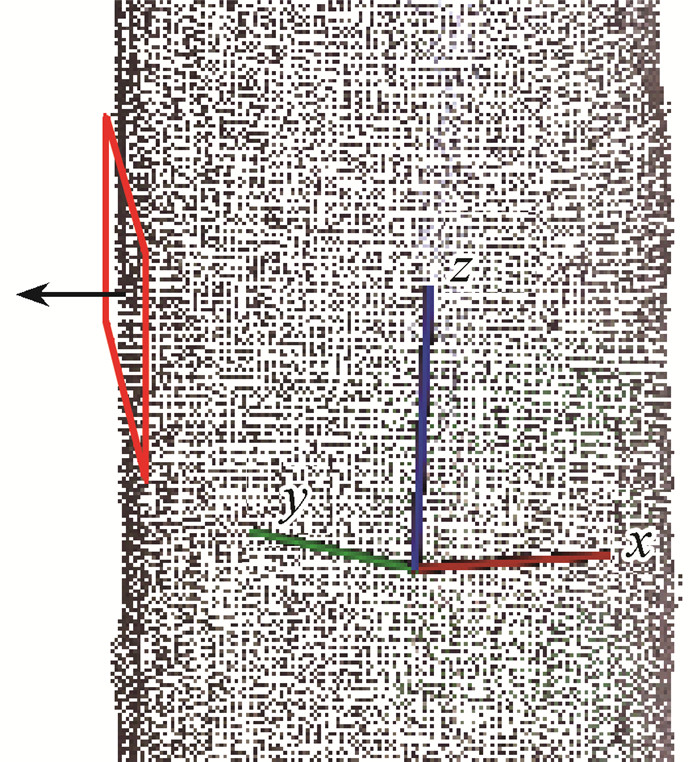图 1 树干点云的切平面 Fig.1 Tangent plane of stem point cloud 图中矩形代表切平面，箭头所示方向为切平面法向量的方向。 The rectangle represents the tangent plane of a point. The arrow direction is the normal vector direction of the tangent plane.

Delaunay近邻点：平面点集上，与点Pi构成一个Delaunay三角形的另外2个顶点称为Pi的Delaunay近邻点。

1.2.2 算法概述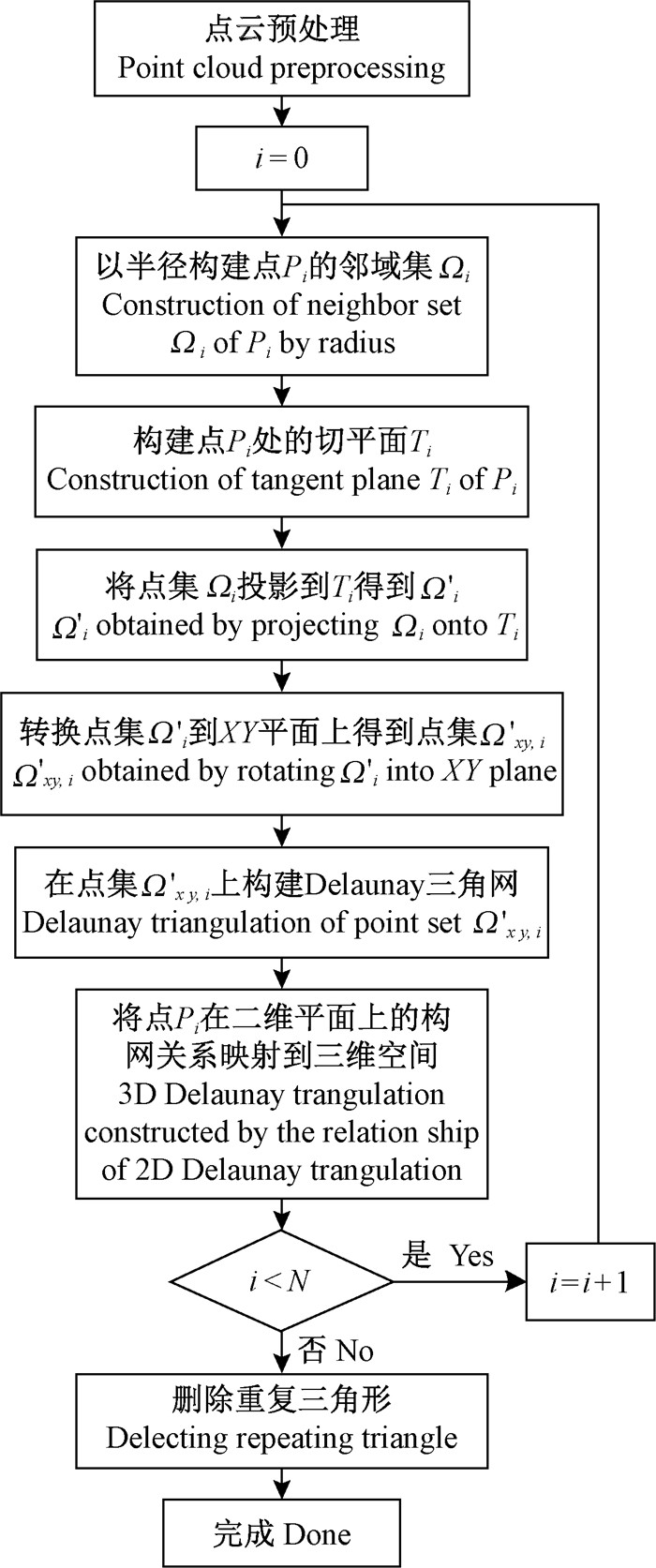图 2 算法流程 Fig.2 The flowchart of the algorithm

1)数据预处理离散噪声点通过给定半径范围内邻域点的数量来判定。当点Pi在邻域半径Rn范围内邻域个数小于Nn时，点Pi就是离散噪声点。

2)邻域集的选择准则对于树干点云集P中的1个点Pi，其邻域点集Ωi决定了与点Pi构成三角形面片的其他顶点。Gopi等(2000)张剑清等(2011)均使用k个近邻点(k-nearest)获取邻域点。受树干表面粗糙性及扫描时受遮挡的影响，点Pi的邻域点分布可能不均匀，有可能出现一侧邻域点密集而另一侧邻域点稀少的情况。在重建表面时，点Pi与其四周的邻域点构成三角形，考虑到与点Pi构成三角形的邻域点的不确定性，很难选定一个合适的k值以适合所有点的表面重建。如图 3所示，当k=5时，蓝色点的邻域点不包括绿色点。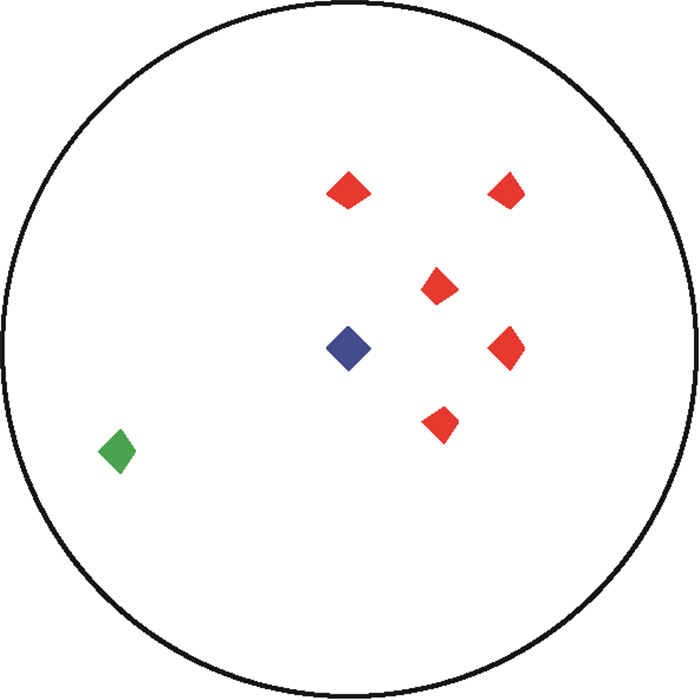图 3 点云密度分布不均匀 Fig.3 The density of point cloud is uneven

 ${\mathit{\Omega }_\mathit{i}}=\left\{ {{P_j}\left| {\left\| {{P_i} - {P_j}} \right\| <{R_s}, {P_i}, {P_j} \in P} \right.} \right\}.$ (2)

3)切平面的简化构建及投影计算切平面法向量的计算以邻域点集Ωi为数据基础。显然，到点Pi距离越近的点，其对树干在点Pi处的切平面的影响越大。张剑清等(2011)以点Pi为中心点构建协方差矩阵计算切平面法向量，并未考虑距离对切平面的影响。本研究采用Cheng等(2006)使用的加权协方差矩阵计算切平面的法向量。定义加权系数为：

 ${w_j}=w\left({\left\| {{P_j} - {P_i}} \right\|/n} \right).$ (3)

 $w\left(x \right)={\left({1 - x} \right)^4}\left({1+4x} \right).$ (4)

 $M=\sum\limits_{{P_i}, {P_j} \in \mathit{\Omega }, j \ne i} {{{\left[ {{w_j}\left({{P_j} - {P_i}} \right)} \right]}^{\rm{T}}}} \left[ {{w_j}\left({{P_j} - {P_i}} \right)} \right].$ (5)

Gopi等(2000)张剑清等(2011)均对法向量的方向进行了归一化处理。对于基于切平面的重建算法，此过程可以省略。

 $\left({q - o} \right){{\hat n}_i}=0.$ (6)

4)投影点集的旋转因投影平面是一个三维空间上的平面，故投影点集Ωi是一个三维空间中的平面点集。因平面Delaunay三角网的构建算法一般都是在二维XY平面上实现的，因此构建三维平面的Delaunay三角网可以有2种方案：(1)实现三维平面上的Delaunay三角网构网算法；(2)将三维平面点集转换到二维XY平面上，在二维XY平面上构建Delaunay三角网。张剑清等(2011)采用第2种方案，通过构建三维平面上的一组正交单位向量将点逐一分解以转换到二维XY平面上，这种方法依赖于正交单位向量的选择且计算较为复杂。

 $R\left(\beta \right){R_x}\left(\alpha \right)=\left[ {\begin{array}{*{20}{c}} {\cos \beta }&{ - \sin \alpha \sin \beta }&{\cos \alpha \sin \beta }\\ 0&{\cos \alpha }&{ - \sin \alpha }\\ {\sin \beta }&{in\alpha \cos \beta }&{\cos \alpha \cos \beta } \end{array}} \right];$ (7)
 $\alpha=\left\{ \begin{array}{l} \arcsin \left[ {{y_i}/{\rm{sqrt}}\left({y_i^2+z_i^2} \right)} \right], y_i^2+z_i^2 \ne 0, \\ 0, y_i^2+z_i^2=0; \end{array} \right.$ (8)
 $\beta=\arcsin \left[ {{x_i}/{\rm{sqrt}}\left({x_i^2+y_i^2+z_i^2} \right)} \right].$ (9)

5)二维Delaunay三角网的构建  Gopi等(2000)张剑清等(2011)均根据Delaunay三角网与Voronoi图的等价性，通过搜索点Pi(点Pi在二维平面上的投影点)的邻近区域的边界来确定Pi的Delaunay近邻点，这种方法计算复杂。本研究使用尤磊等(2016)提出的以优先点为中心的Delaunay构网算法在XY平面上构建点集Ωxy, i的Delaunay三角网。根据Delaunay三角网中点Pi与其他点的连接关系得到Delaunay近邻点。

6)三维Delaunay三角网的构建  将点Pi与其Delaunay近邻点的连接关系映射到树干点云上，构成以Pi为顶点的多个三角形面片，完成点Pi的表面重建。

7)算法参数列表  算法需要使用的参数如表 1所示。8)表面模型的定量评价  树皮的粗糙性会通过树干点云间接反映在重建的树干表面上。在相同位置，从重建的树干表面上提取的直径与使用围尺测量的直径越接近，重建表面的效果越好。由此，本研究提出一种定量化评价树干重建表面模型的方法：根据围尺测量的直径与从重建树干表面上对应位置处提取直径的差异来评价树干的重建表面模型。对应的评价指标如下：

 $MAPE=100{\rm{ \times }}\frac{1}{n}\sum\limits_{i=1}^n {\left| {{D_i} - {{\hat D}_i}} \right|/{D_i};}$ (10)
 ${\rm{RMSE=}}\sqrt {\frac{1}{n}\sum\limits_{i=1}^n {{{\left({{D_i} - {{\hat D}_i}} \right)}^2}} }.$ (11)

2 结果与分析

2.1 与经典算法对比试验

Gopi等(2000)张剑清等(2011)描述的切平面重建算法为经典算法，本文的算法为改进算法。因切平面邻域点集的选择对重建表面的效果影响较大，因此本文仅以邻域点集的不同来体现经典算法与改进算法的差异。经典算法以邻域点个数k选择邻域点集，改进算法以邻域半径Rs选择邻域点集。对于采用默认规格下采样后的树干点云，因下采样后1个栅格中只有1个点，且因1个三维栅格最多与8个三维栅格相邻，因此经典算法以k=8选择邻域点集；根据上述，改进算法以Rs=1 cm选择邻域点集。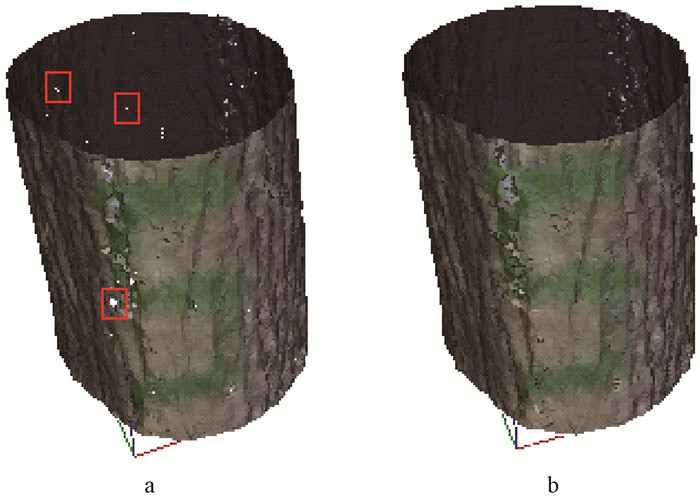图 4 不同算法重建的表面 Fig.4 Surface reconstructed by different algorithms a.经典算法Classic; b.改进算法Improved.

2.2 改进算法的表面重建试验结果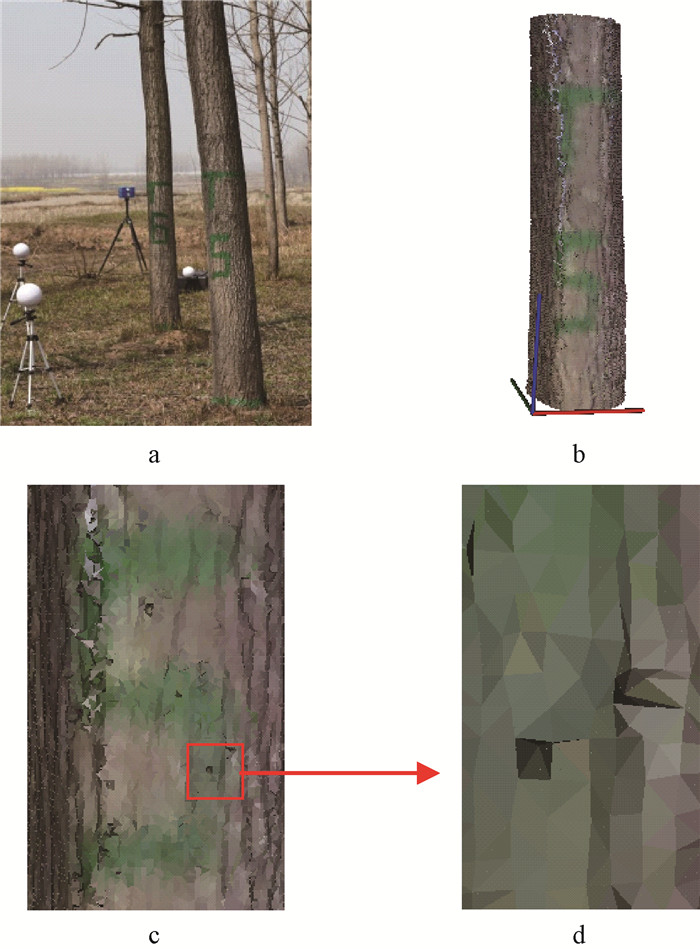图 5 一段杨树树干点云及其重建的表面 Fig.5 A part of Populussp. stem point cloud and reconstructed surface a.外业扫描时照片Picture of the field working; b.重建的表面Reconstructed surface; c.局部表面Partial reconstructed surface; d.表面三角面片Triangular patch of reconstructed surface.下同The same below.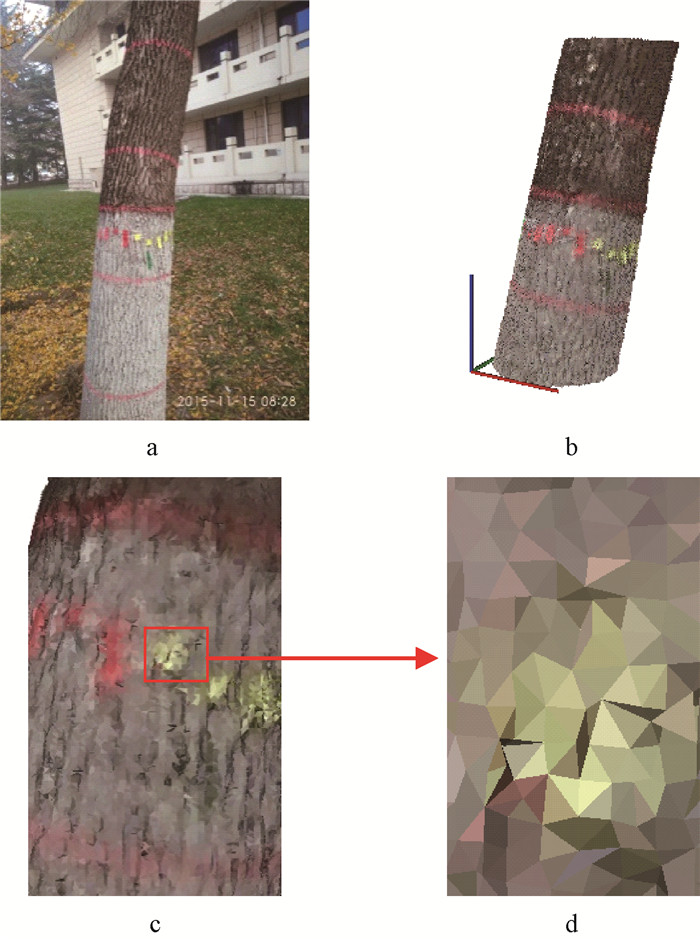图 6 一段洋白蜡树干点云及其重建的表面 Fig.6 A part of Fraxinus pennsylvanica stem point cloud and reconstructed surface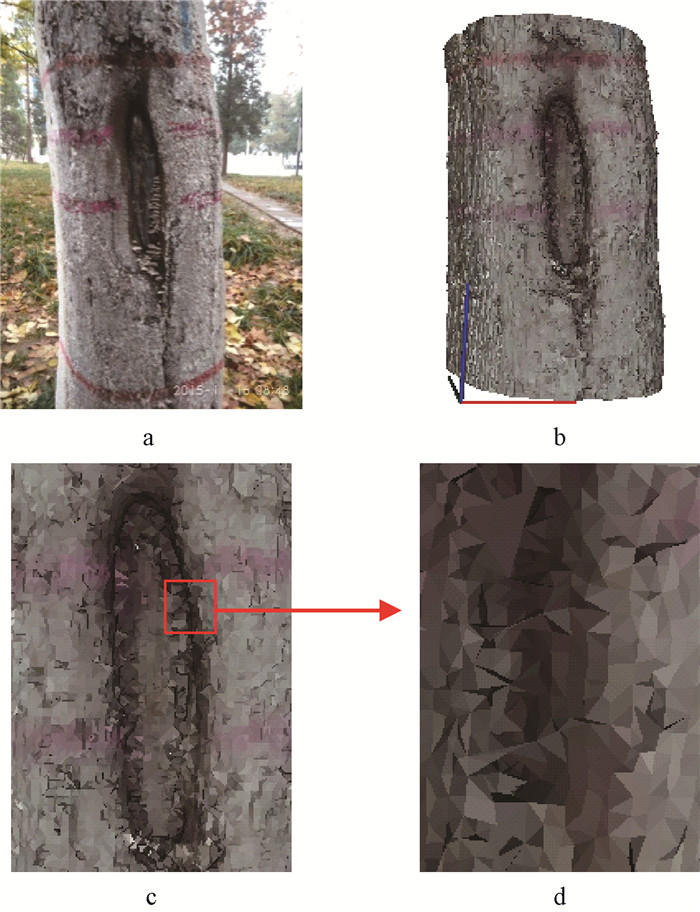图 7 一段大叶白蜡树干点云及其重建的表面 Fig.7 A part of Fraxinus chinensisvar.rhynchophylla stem point cloud and reconstructed surface
2.3 重建表面的定量评价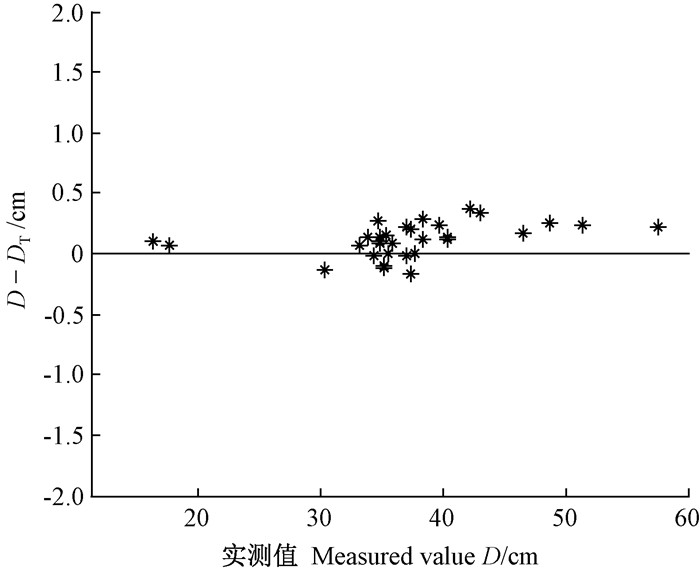图 8 从重建表面上提取直径与实测直径的差值 Fig.8 The diameter differences between extracting from reconstructed surface and field measurement

3 讨论

4 结论

 [] 高士增, 张怀清, 刘闽, 等. 2013. 树木枝干Delaunay三角网格构建技术. 西南林业大学学报 , 33 (3) : 62–68. ( Gao S Z, Zhang H Q, Liu M, et al.2013. Constructing technology of tree branches Delaunay triangulation. Journal of Southwest Forestry University , 33 (3) : 62–68. [in Chinese] ) [] 黄洪宇, 陈崇成, 邹杰, 等. 2013. 基于地面激光雷达点云数据的单木三维建模综述. 林业科学 , 49 (4) : 123–130. ( Huang H Y, Chen C C, Zou J, et al.2013. Tree geometrical 3D modeling from terrestrial laser scanned point clouds: a review. Scientia Silvae Sicicae , 49 (4) : 123–130. [in Chinese] ) [] 王晓辉, 黄洪宇, 陈崇成, 等. 2014. 基于激光点云的树木三维几何建模系统的设计与实现. 福州大学学报:自然科学版 , 42 (5) : 705–712. ( Wang X H, Huang H Y, Chen C C, et al.2014. Design and implementation of 3D geometrucal tree modeling system based on terrestrial laser scanned point cloud data. Journal of Fuzhou University:Natural Science Edition , 42 (5) : 705–712. [in Chinese] ) [] 尤承业. 2004. 解析几何. 北京: 北京大学出版社 . ( You C Y. 2004. Analytic geometry. Beijing: Beijing University Press . [in Chinese] ) [] 尤磊, 唐守正, 宋新宇. 2016. 以优先点为中心的Delaunay三角网生长算法. 中国图象图形学报 , 21 (1) : 60–68. ( You L, Tang S Z, Song X Y.2016. Growth algorithm centered on priority for constructing the Delaunay triangulation. Journal of Image and Graohics , 21 (1) : 60–68. [in Chinese] ) [] 袁方, 唐杰, 武港山. 2011. 一种基于三维Delaunay三角化的曲面重建算法. 计算机技术与发展 , 21 (10) : 14–18. ( Yuan F, Tang J, Wu G S.2011. A geometric spread approach of 3-D reconstruction. Computer Technology and Development , 21 (10) : 14–18. [in Chinese] ) [] 张剑清, 李彩林, 郭宝云. 2011. 基于切平面投影的散乱数据点快速曲面重建算法. 武汉大学学报:信息科学版 , 36 (7) : 757–762. ( Zhang J Q, Li C L, Guo B Y.2011. A fast surface reconstruction algorithm for unorganized points based on tagent plane projection. Geomatics and Information Science of Wuhan University , 36 (7) : 757–762. [in Chinese] ) [] 朱德海. 2012. 点云库PCL学习教程. 北京: 北京航空航天大学出版社 . ( Zhu D H. 2012. Point cloud library PCL. Beijing: Beihang University Press . [in Chinese] ) [] Cheng Z, Zhang X, Fourcaud T.2006. Tree skeleton extraction from a single range image//Plant Growth Modeling and Applications. Second International Symposium on IEEE : 274–281. [] De Berg M, Van Krevedl M, Overmars M, et al. 2009. Computational geometry: algorithms and applications. 3rd ed. New York:Springer-Cerlag. [] Gopi M, Krishnan S, Silva C T.2000. Surface reconstruction based on lower dimensional localized Delaunay triangulation. Computer Graphics Forum , 19 (3) : 467–478. DOI:10.1111/1467-8659.00439 [] Gorte B, Pfeifer N.2004a. Structuring laser-scanned trees using 3D mathematical morphology. International Archives of Photogrammetry and Remote Sensing , 35 (B5) : 929–933. [] Gorte B, Winterhalder D.2004b. Reconstruction of laser-scanned trees using filter operations in the 3D raster domain. International Archives of Photogrammetry, Remote Sensing and Spatial Information Sciences , 36 (Part 8) : W2. [] Hackenberg J, Spiecker H, Calders K, et al.2015. SimpleTree-an efficient open source tool to build tree models from TLS clouds. Forests , 6 (11) : 4245–4294. [] Pfeifer N, Gorte B, Winterhalder D.2004a. Automatic reconstruction of single trees from terrestrial laser scanner data. Proceedings of 20th ISPRS Congress : 114–119. [] Pfeifer N, Winterhalder D.2004b. Modelling of tree cross sections from terrestrial laser scanning data with free-form curves. International Archives of Photogrammetry, Remote Sensing and Spatial Information Sciences , 36 (Part 8) : W2. [] Rusu R B, Cousins S.2011. 3D is here: point cloud library (PCL)//Robotics and automation (ICRA). 2011 IEEE International Conference on IEEE, 1-4. [] Xu H, Gossett N, Chen B.2007. Knowledge and heuristic-based modeling of laser-scanned trees. ACM Transactions on Graphics (TOG) , 26 (4) : 19. DOI:10.1145/1289603